Function Repository Resource:

# PermutationIndex

Give the lexicographic index of a permutation

Contributed by: Ed Pegg Jr
 ResourceFunction["PermutationIndex"][perm] gives the lexicographic index of permutation perm.

## Details and Options

Permutations are considered to be in normal sorted order.
Lehmer codes are generated as an intermediary step.
The generating algorithm for Lehmer codes is as follows: For each number in the permutation, count how many subsequent values it exceeds.

## Examples

### Basic Examples (2)

Return the index of a permutation:

 In:=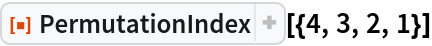Out=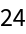The first permutation of a given length has index 1:

 In:=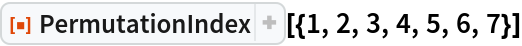Out=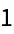### Scope (4)

Some large permutations:

 In:=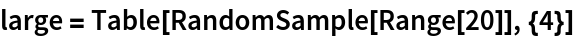Out=Large permutations can be indexed:

 In:=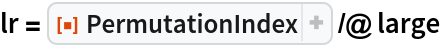Out=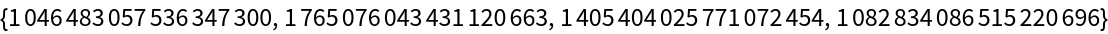Terms don’t necessarily need to be positive or integer:

 In:=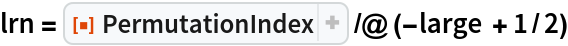Out=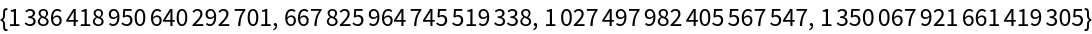The indices of permutations and negative permutations are balanced:

 In:=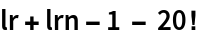Out=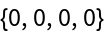### Neat Examples (1)

Differences of indices of even permutations:

 In:=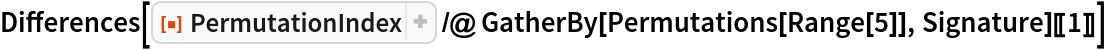Out=## Version History

• 1.0.0 – 20 December 2019